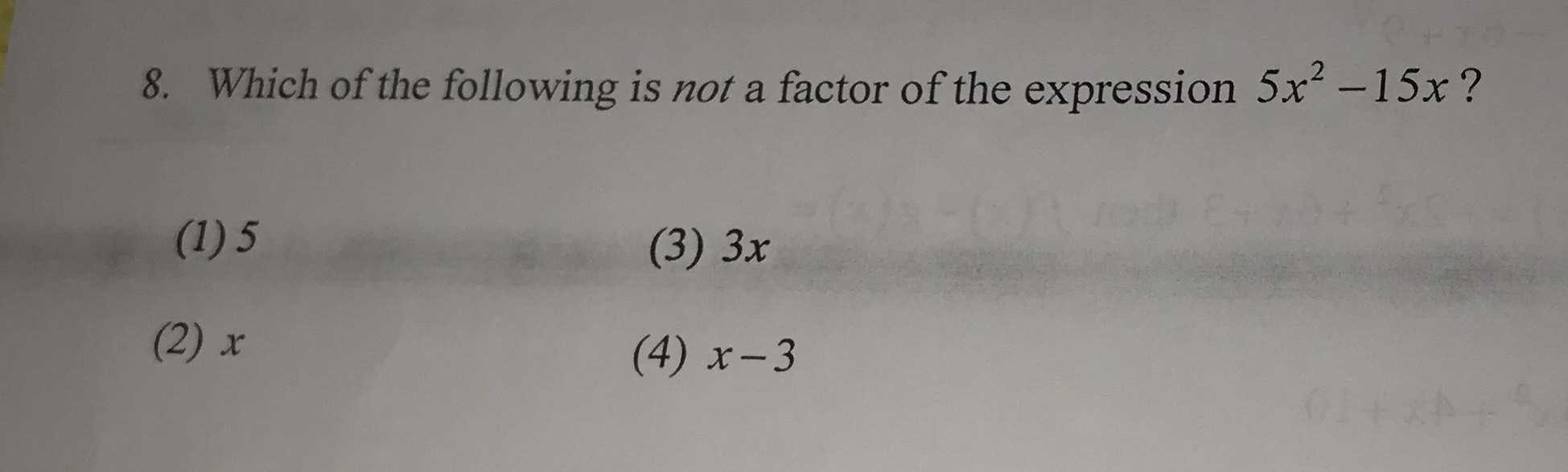### ¿Todavía tienes preguntas de matemáticas?

Pregunte a nuestros tutores expertos
Algebra
Pregunta8. Which of the following is not a factor of the expression $$5 x ^ { 2 } - 15 x$$ ?

(1) $$5$$ (3) $$3 x$$

(2) $$x$$ (4) $$x - 3$$